Скачать презентацию Option pricing with sparse grid quadrature JASS 2007

53c47717feb9eb534526d8fb19239567.ppt

• Количество слайдов: 32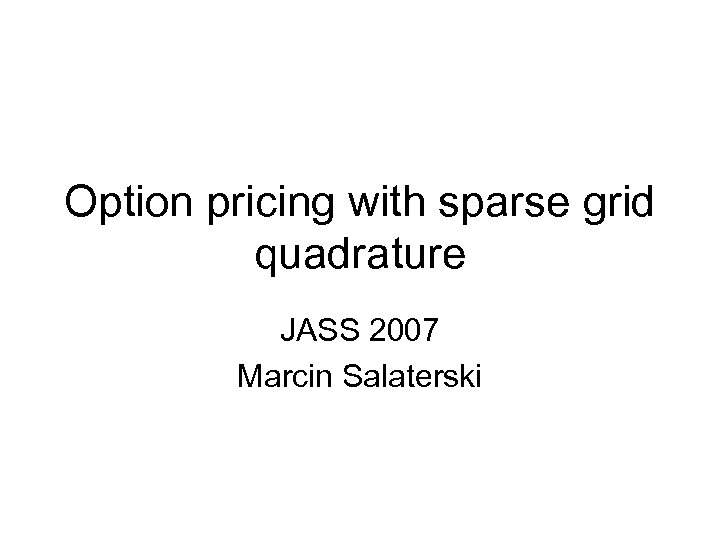Option pricing with sparse grid quadrature JASS 2007 Marcin Salaterski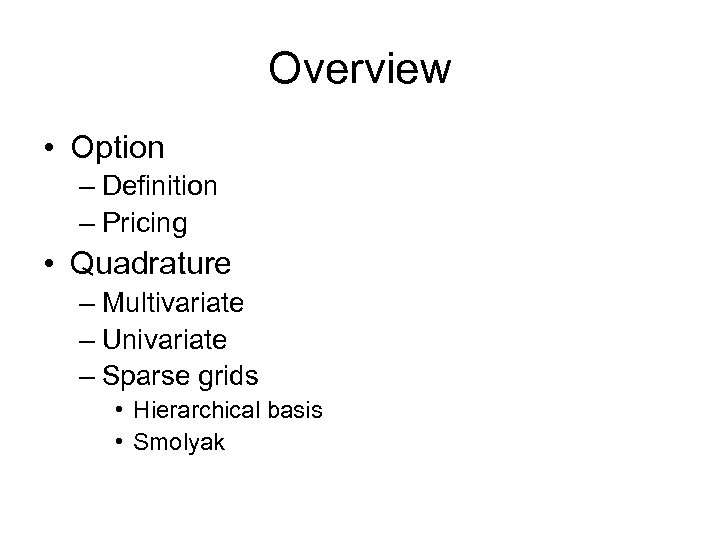Overview • Option – Definition – Pricing • Quadrature – Multivariate – Univariate – Sparse grids • Hierarchical basis • Smolyak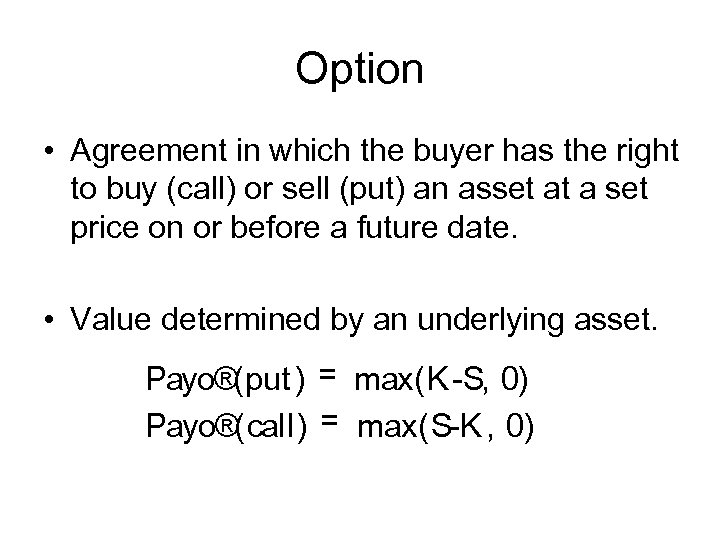Option • Agreement in which the buyer has the right to buy (call) or sell (put) an asset at a set price on or before a future date. • Value determined by an underlying asset. Payo®(put ) = max(K S, 0) Payo®(call) = max(S K , 0)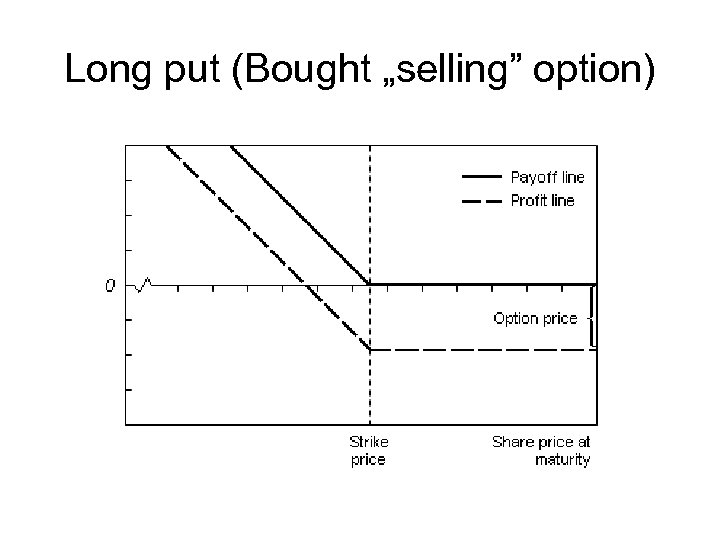Long put (Bought „selling” option)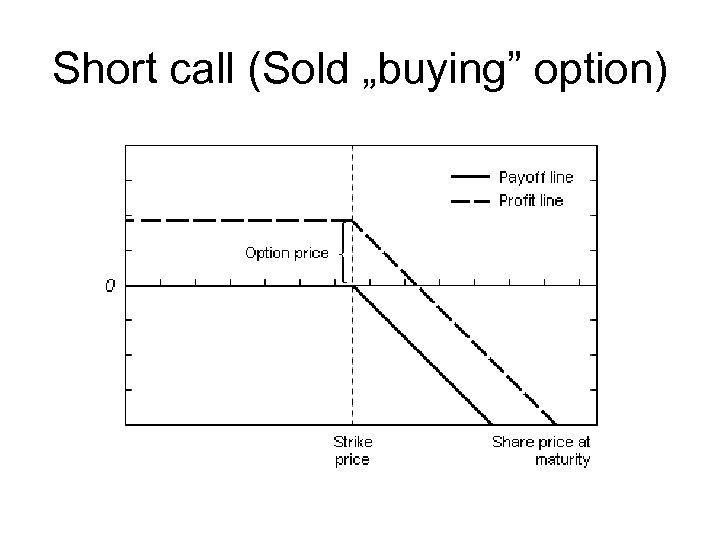Short call (Sold „buying” option)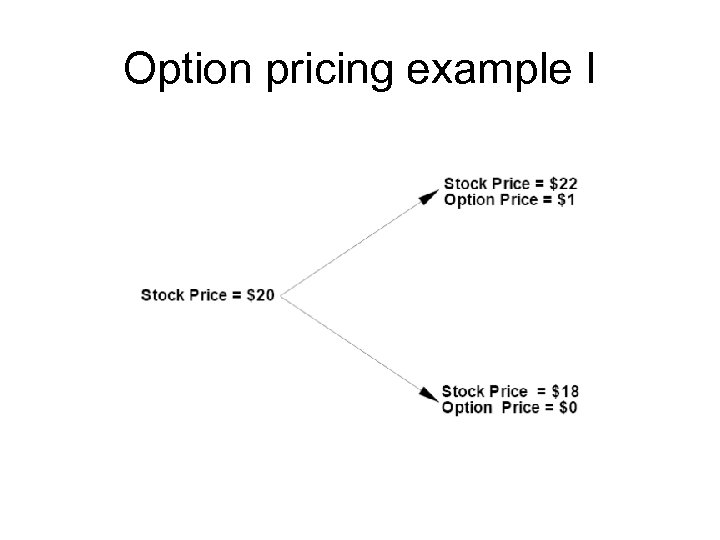Option pricing example I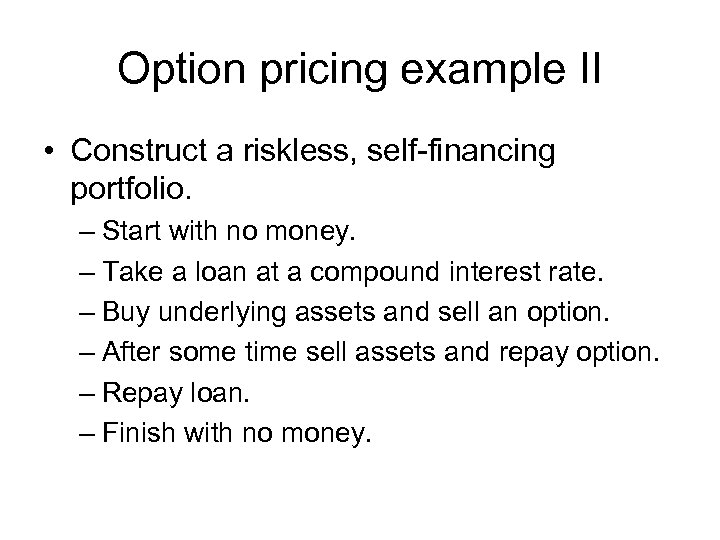Option pricing example II • Construct a riskless, self financing portfolio. – Start with no money. – Take a loan at a compound interest rate. – Buy underlying assets and sell an option. – After some time sell assets and repay option. – Repay loan. – Finish with no money.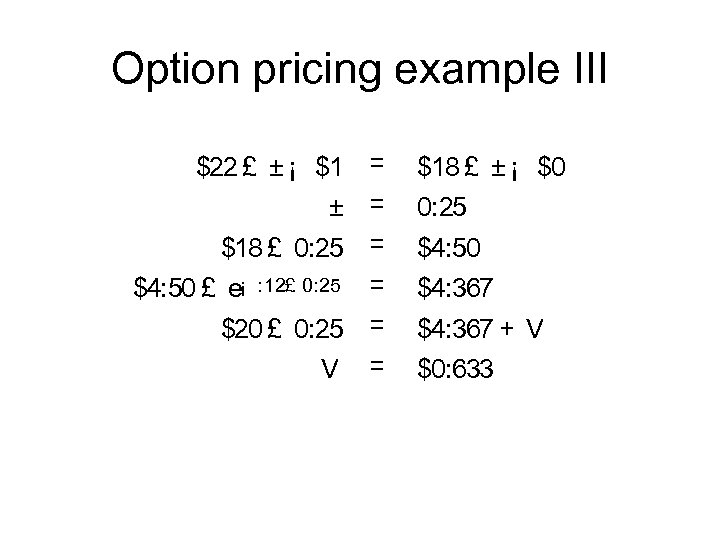Option pricing example III \$22 £ ± ¡ \$1 = ± = \$18 £ 0: 25 = \$4: 50 £ e¡ : 12£ 0: 25 = \$20 £ 0: 25 = V = \$18 £ ± ¡ \$0 0: 25 \$4: 50 \$4: 367 + V \$0: 633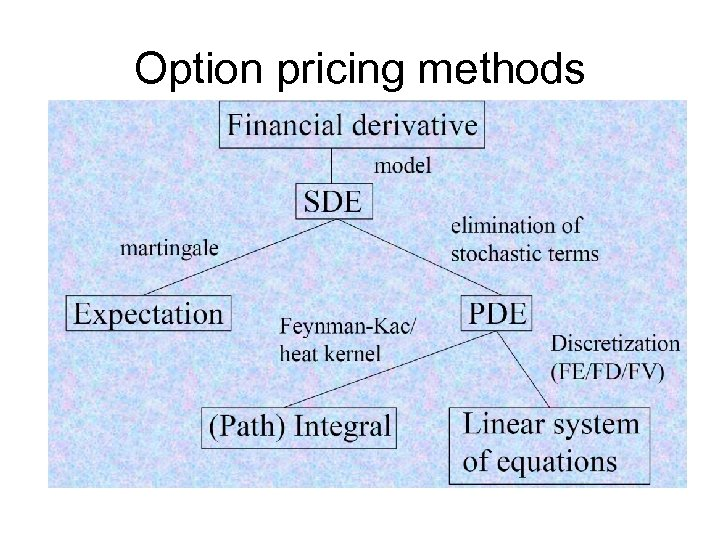Option pricing methods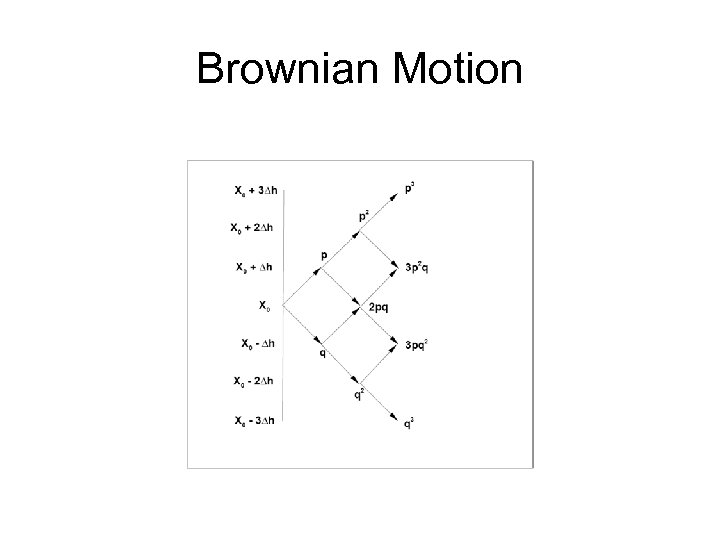Brownian Motion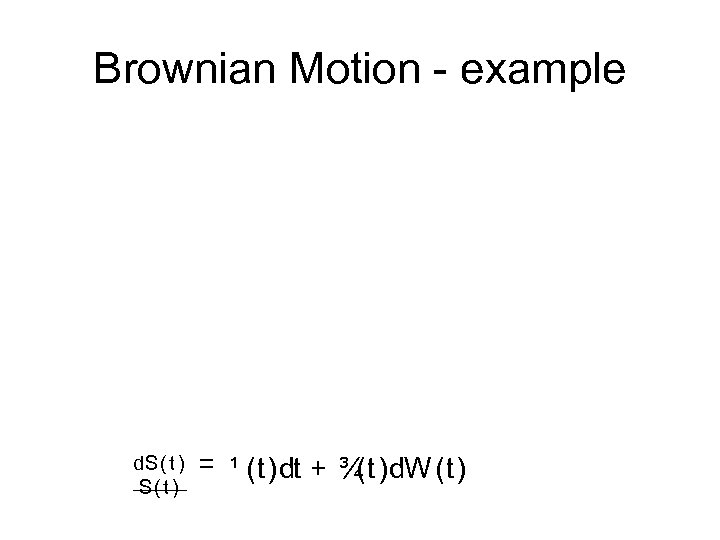Brownian Motion example d. S( t ) = ¹ (t)dt + ¾ (t)d. W (t)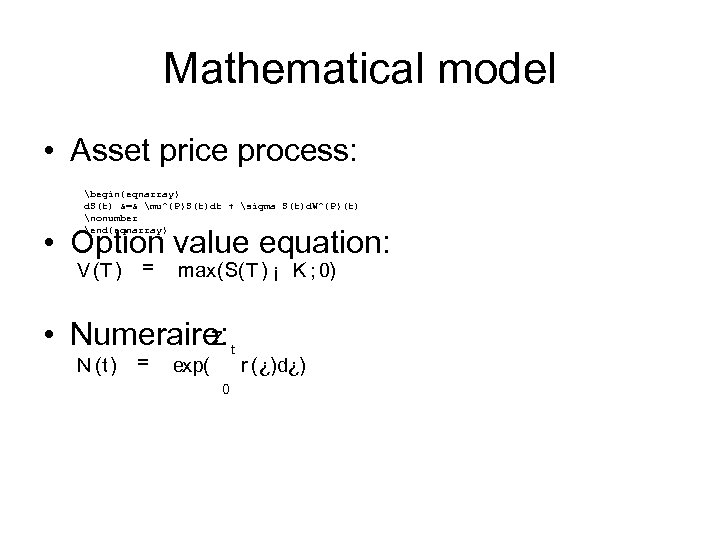Mathematical model • Asset price process: begin{eqnarray} d. S(t) &=& mu^{P}S(t)dt + sigma S(t)d. W^{P}(t) nonumber end{eqnarray} • Option value equation: V (T ) = max(S(T ) ¡ K ; 0) Z • Numeraire: t N (t) = exp( 0 r (¿)d¿)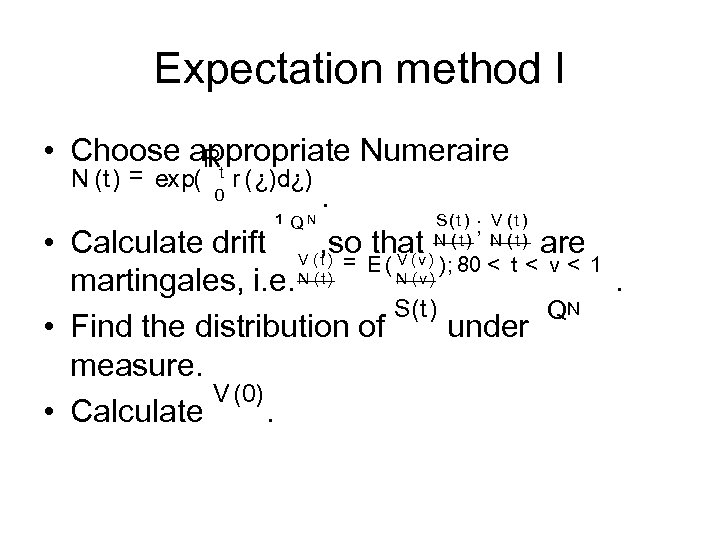Expectation method I • Choose appropriate Numeraire Rt N (t) = exp( r (¿)d¿) 0. S( t ) ; V ( t ) ¹Q • Calculate drift V (, so thatv)N ( t ) are t ) = E ( V ( ); 80 < t < v < 1 N (v) martingales, i. e. N ( t ). S(t) QN • Find the distribution of under measure. V (0) • Calculate. N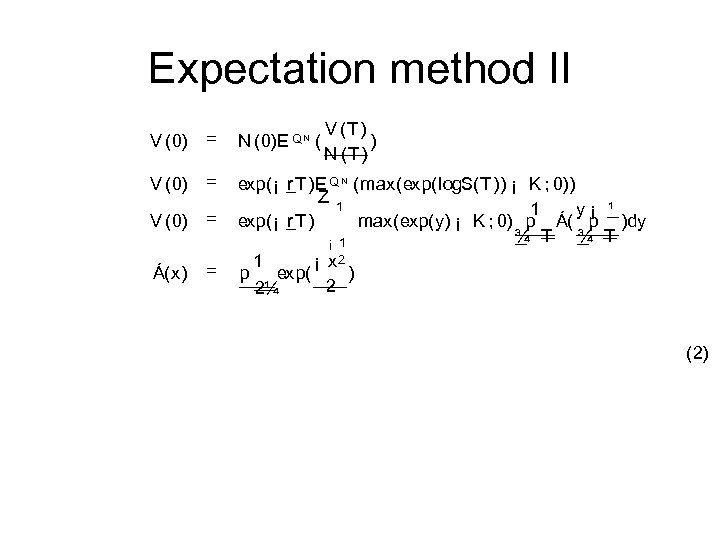Expectation method II V (T ) ) N (T ) V (0) = N (0)E Q N ( V (0) = Á(x) = exp(¡ r T )E Q N (max(exp(log. S(T )) ¡ K ; 0)) Z 1 1 y¡ ¹ exp(¡ r T ) max(exp(y) ¡ K ; 0) p Á( p )dy ¾ T ¡ 1 1 ¡ x 2 p exp( ) 2 2¼ (2)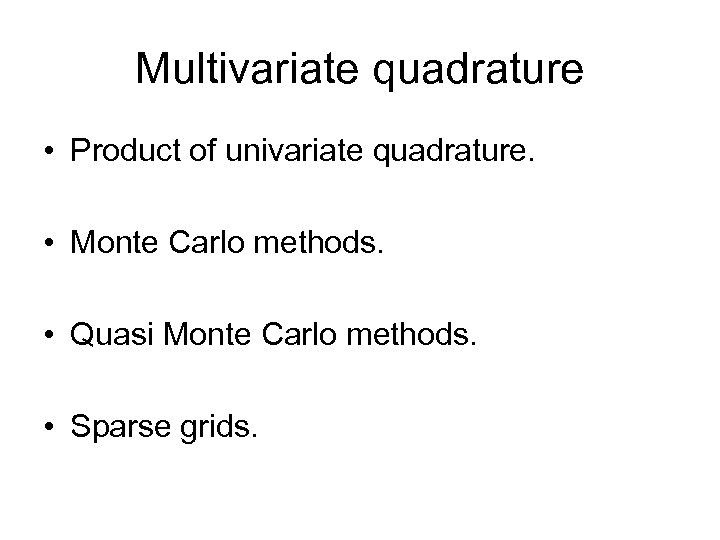Multivariate quadrature • Product of univariate quadrature. • Monte Carlo methods. • Quasi Monte Carlo methods. • Sparse grids.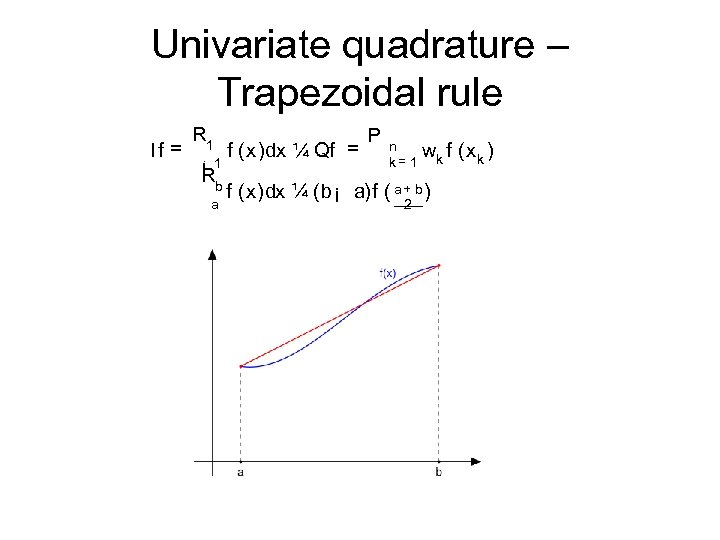Univariate quadrature – Trapezoidal rule If = R 1 ¡ 1 Rb a f (x)dx ¼ Qf = P n k= 1 wk f (x k ) f (x)dx ¼ (b¡ a)f ( a+ b ) 2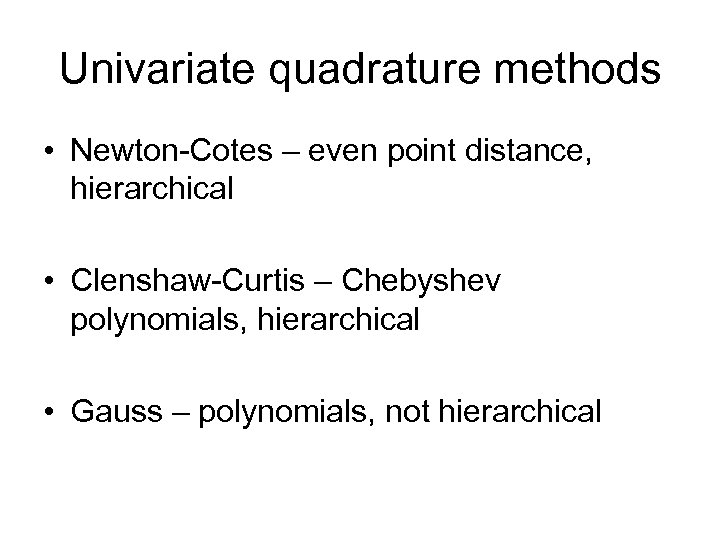Univariate quadrature methods • Newton Cotes – even point distance, hierarchical • Clenshaw Curtis – Chebyshev polynomials, hierarchical • Gauss – polynomials, not hierarchical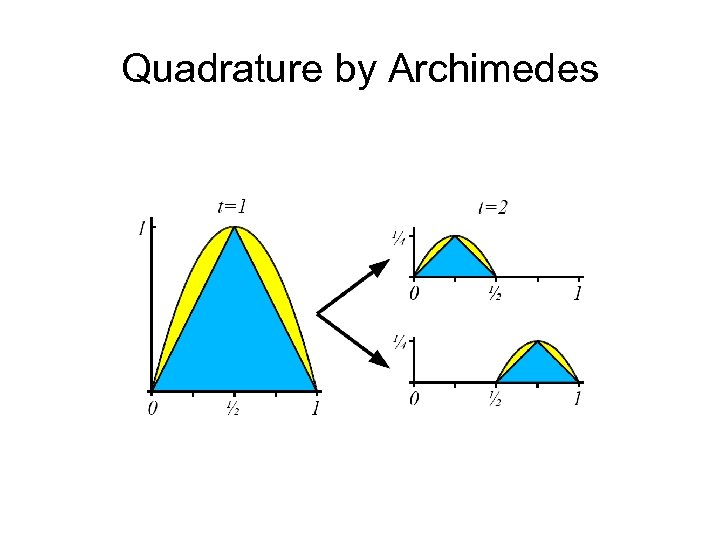Quadrature by Archimedes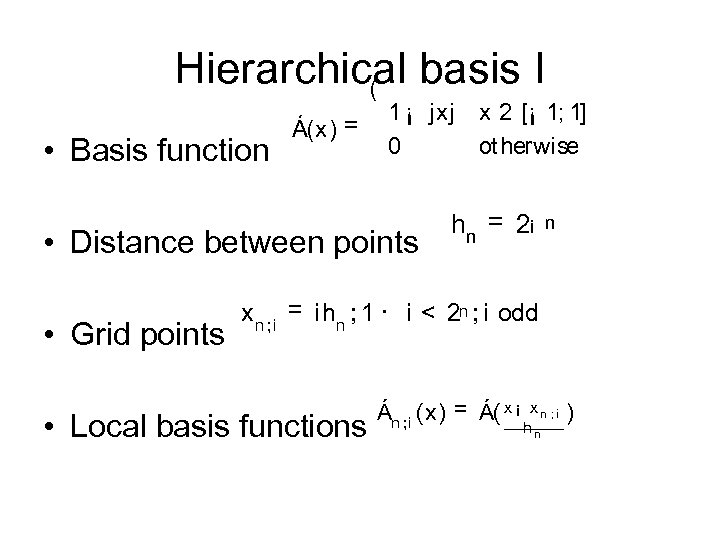Hierarchical basis I ( • Basis function Á(x) = 1 ¡ jxj 0 • Distance between points • Grid points x 2 [¡ 1; 1] ot herwise h n = 2¡ n x n ; i = i hn ; 1 · i < 2 n ; i odd • Local basis functions Án ; i (x) = Á( x ¡ xn ; i hn )Hierarchical basis II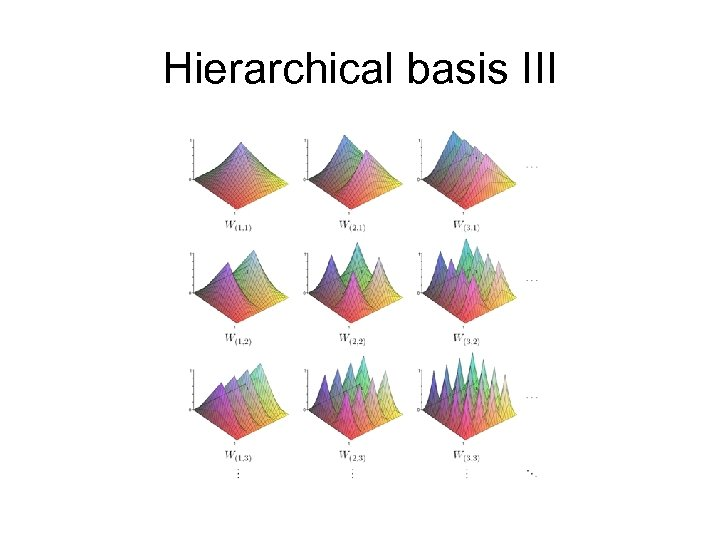Hierarchical basis III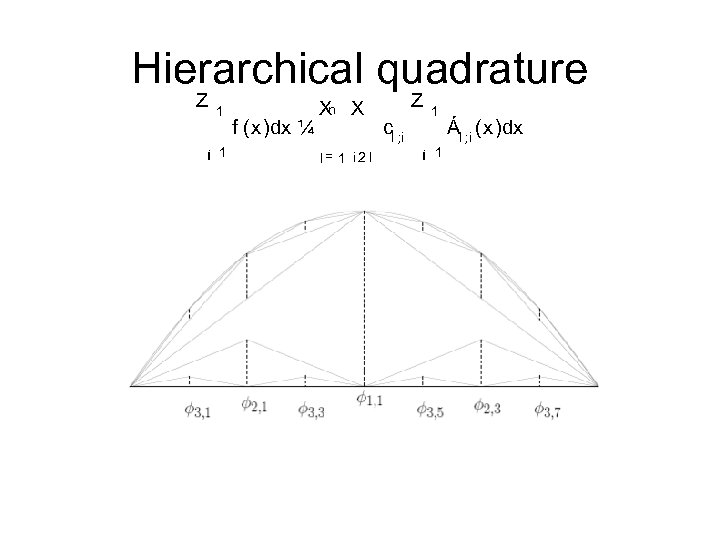Hierarchical quadrature Z 1 ¡ 1 f (x)dx ¼ Xn X l= 1 i 2 I Z cl ; i 1 ¡ 1 Ál ; i (x)dx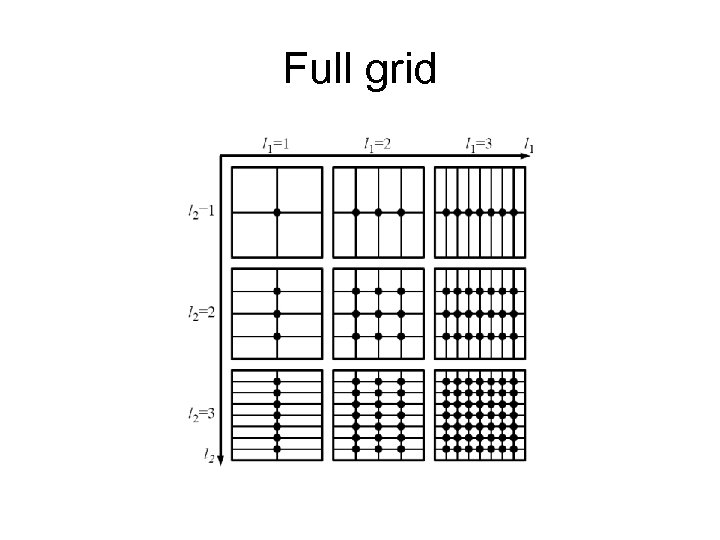Full grid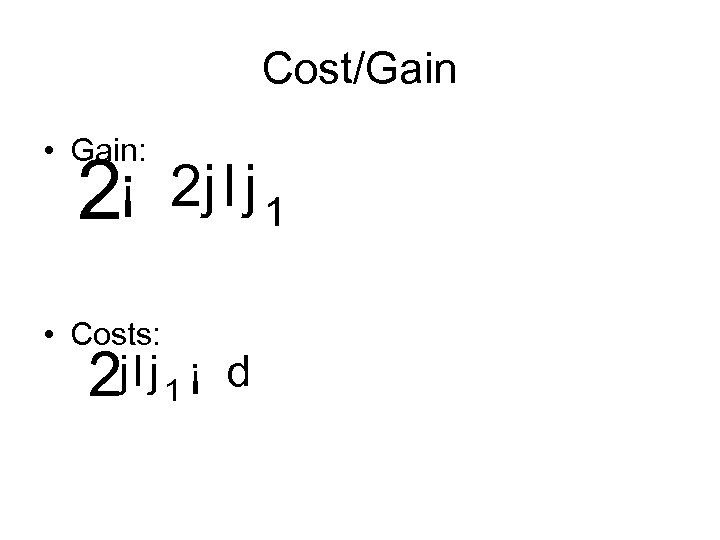Cost/Gain • Gain: 2¡ • Costs: 2 j l j 1 ¡ d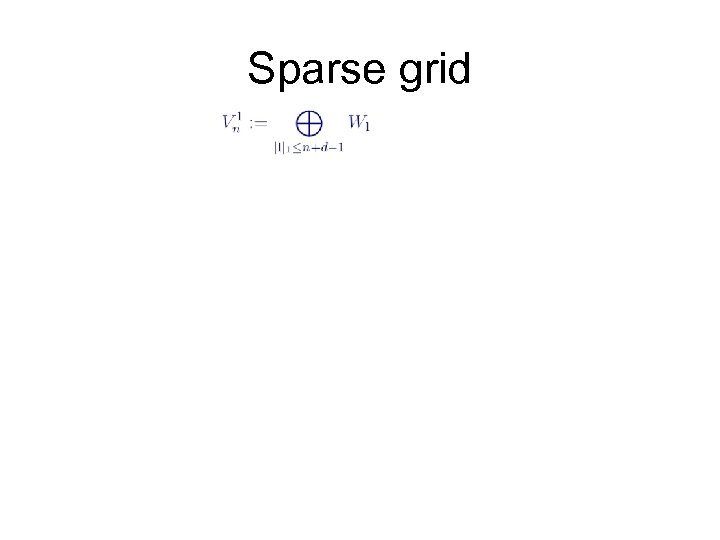Sparse grid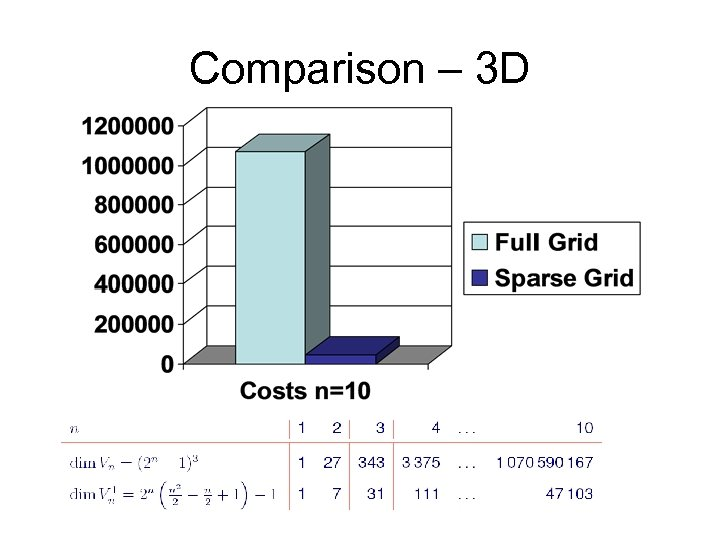Comparison – 3 D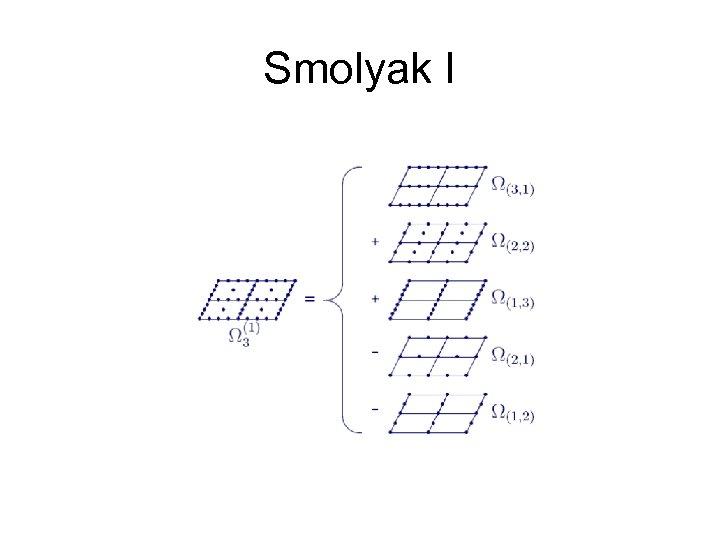Smolyak I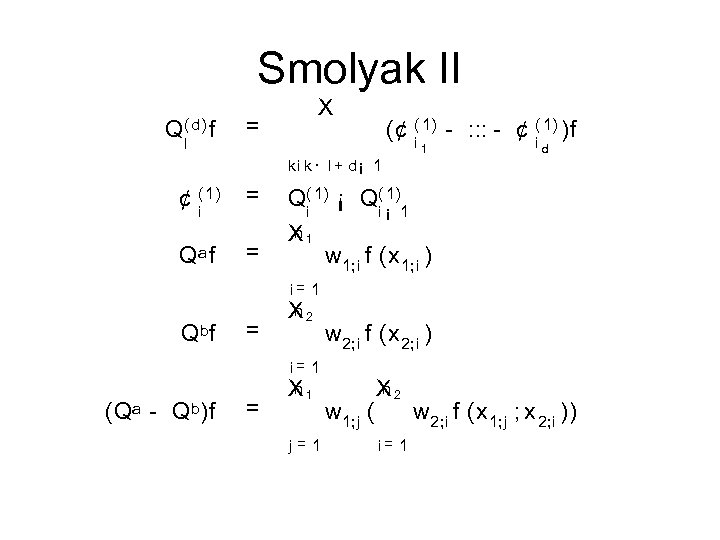Smolyak II Q( d) f l = X (¢ ( 1) : : : ¢ ( 1) )f i i 1 d k i k· l + d¡ 1 ¢ ( 1) i = Qa f = Qbf (Qa Qb)f = = 1) Q( 1) ¡ Q( ¡ 1 i i n X 1 w 1; i f (x 1; i ) i= 1 n X 2 i= 1 n X 1 j=1 w 2; i f (x 2; i ) w 1; j ( n X 2 i= 1 w 2; i f (x 1; j ; x 2; i ))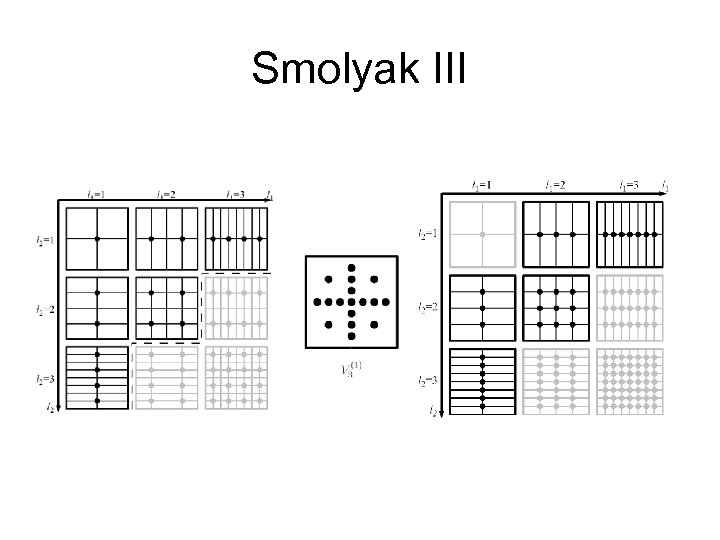Smolyak III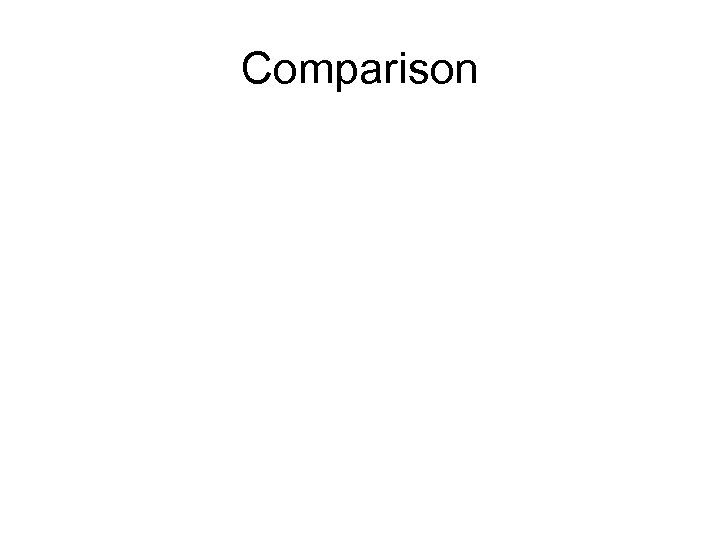Comparison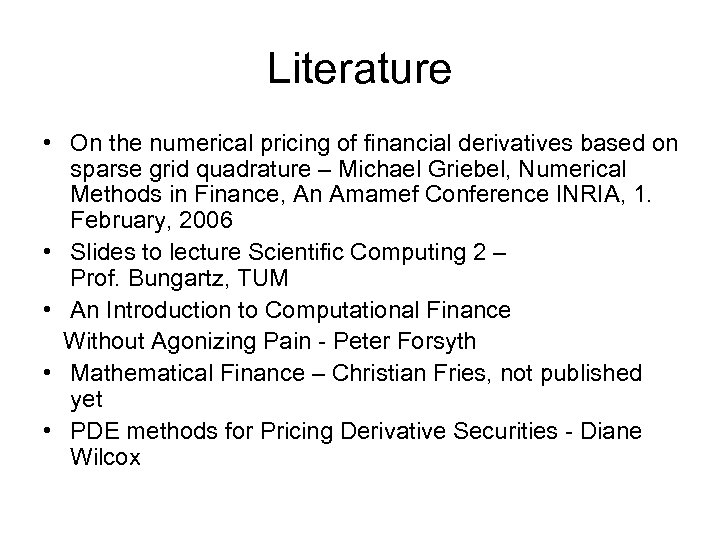Literature • On the numerical pricing of financial derivatives based on sparse grid quadrature – Michael Griebel, Numerical Methods in Finance, An Amamef Conference INRIA, 1. February, 2006 • Slides to lecture Scientific Computing 2 – Prof. Bungartz, TUM • An Introduction to Computational Finance Without Agonizing Pain Peter Forsyth • Mathematical Finance – Christian Fries, not published yet • PDE methods for Pricing Derivative Securities Diane WilcoxThank you !